# Progression

12, 60, -300,1500 need next 2 numbers of pattern

Result

a5 =  7500
a6 =  37500

#### Solution:Leave us a comment of example and its solution (i.e. if it is still somewhat unclear...):

Showing 0 comments:Be the first to comment!## Next similar examples:

1. Probably memberLook at the series 2,6,25,96,285, ? What number should come next?
2. Gauss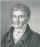Help little C.F. Gauss sum all the integers from 1 to 400.
3. Piano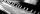If Suzan practicing 10 minutes at Monday; every other day she wants to practice 2 times as much as the previous day, how many hours and minutes will have to practice on Friday?
4. Eight palm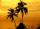There grows 8 palms by the sea. At the first sitting one parrot, on second two, on third sits four parrots on each other twice the previous parrots sitting on a previous palm. How many parrots sitting on eighth palm?
5. Geometric progression 2There is geometric sequence with a1=5.7 and quotient q=-2.5. Calculate a17.
6. Six termsFind the first six terms of the sequence a1 = -3, an = 2 * an-1
7. Three-digitHow many three-digit natural numbers do not have the number 7?
8. Basket of fruitIn six baskets, the seller has fruit. In individual baskets, there are only apples or just pears with the following number of fruits: 5,6,12,14,23 and 29. "If I sell this basket," the salesman thinks, "then I will have just as many apples as a pear." Which
9. Two unknown numbersOne number is 2 less than a second number. Twice the second number is 14 less than 5 times the first. Find the two numbers.
10. Balls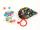Michal said to Martin: give me one ball and I'll have twice as you. Martin said: give me 4 and we will have equally. How many balls each have?
11. Cows and calves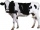There are 168 cows and calves in the cowshed. Cows are in nine stalls and calves in four stalls. Same count cows are in every cow stall and three more in each calf stall than in a cow stall. What is the capacity of the stalls for cows and what for calves?
12. Toy carsPavel has a collection of toy cars. He wanted to regroup them. But in the division of three, four, six, and eight, he was always one left. Only when he formed groups of seven, he divided everyone. How many toy cars have in the collection?
13. Theorem proveWe want to prove the sentense: If the natural number n is divisible by six, then n is divisible by three. From what assumption we started?
14. CandiesIf Alena give Lenka 3 candy will still have 1 more candy. If Lenka give Alena 1 candy Alena will hame twice more than Lenka. How many candies have each of them?
15. AntennasIf you give me two antennas will be same. If you give me again your two antenna I have a 5× so many than you. How many antennas have both mans?
16. The diceWhat is the probability of events that if we throw a dice is rolled less than 6?
17. Three friendsThe three friends spent 600 KC in a teahouse. Thomas paid twice as much as Paul. Paul a half less than Zdeněk. How many each paid?